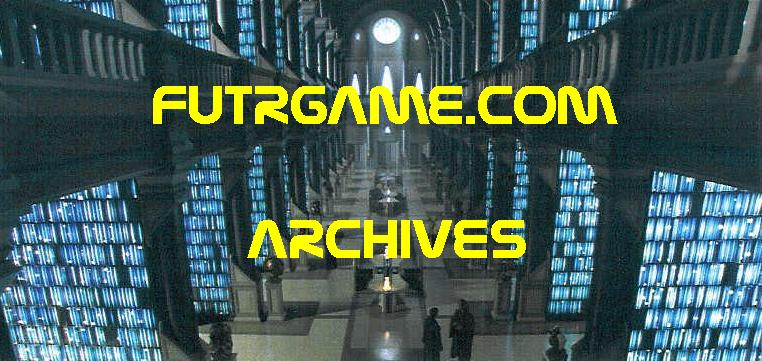gridLINK
WEBSITES USING A 3-COORDINATE SYSTEM
TIME, STRANGENESS, & VIRTUAL CHARACTERISTICS

X = TIME CONTINUUM
0 = NOW = 0
5 = NEAR = 5
10 = FAR FUTURE = 10
Y = STRANGENESS CONTINUUM
0 = NORMAL = 0
5 = MIDWAY = 5
10 = WEIRD = 10
Z = REALITY CONTINUUM
0 = REAL = 0
5 = MIDWAY = 5
10 = VIRTUAL = 10
X Y Z
\ alphaLINK##### Last Update May, 2003